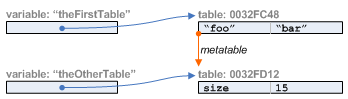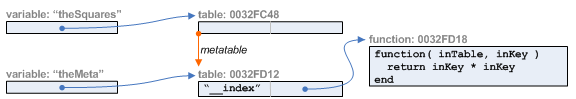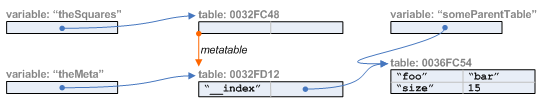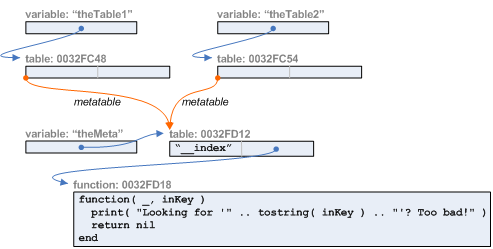# Referenced Values, Metatables, and Simple Inheritance

The nature of variables and values in Lua, what 'metatables' are, and how they can be used to create inheritance. See Learning Lua for more resources.

## Variables, Fields, and Values

First-time scripters often think of variables as "holding" or "containing" values. This is true for the simple values types—nil, boolean, number, and string—but is not true for types of values that are 'objects': table, function, and userdata. For these types of values, variables (and table fields) contain references to the object.variables and fields contain some value types directly, others as references

You can have more than one variable or table field referring to the same object. For example, in the above diagram the variable phleep is referring to the same table as runData.cats. Modifying the object affects all references.

``````print( phleep.alive )
--> true

runData.cats[ 1 ].alive = "I'm not dead yet!"
print( phleep.alive )

The code sample above shows the table field changing from a boolean value of true to a string value of "I'm not dead yet!". Fields, like variables, do not have a particular type. Instead, they can contain any value; it is that value that has a type.

## Tables and Metatables and Inheritance

Each table in Lua can optionally have a 'metatable' associated with it.

``````local theFirstTable = { foo = "bar" }
local theOtherTable = { size = 15 }

setmetatable( theFirstTable, theOtherTable )``````

In the above, we call theOtherTable a metatable. Metatables are not special tables; they are normal tables which just happen to be associated with another table. Nothing about the table changes when it is used as a metatable.one table used as the metatable of another

Metatables can be used to associate extra data with a table, perhaps when you don't want the extra data to show up in the key list for the original table. Metatables are primarily used, however, to cause Lua to perform some nice magic. When a table has a metatable, and that metatable has certain 'special' keys, extra functionality is gained. (See Lua Metatable Events for the full list of keys and their effect.)

The most common special metatable key is __index. (That's two underscores up front.) It can have a value that is a function, or a value that is a table.

### __index as a function

If a table has a metatable, and that metatable has a key named "__index" whose field value is a function, that function will be called (with the original table and the key passed as parameters) whenever Lua code asks for a key from the original table and that key doesn't exist. Whatever value that function returns will be returned for the key.

That was a really long description. Let's see this in action:using the __index 'metaevent' to call a function for unknown keys
``````-- An empty table
theSquares = { }
print( theSquares[ 13 ] )
--> nil

theMeta = { }
setmetatable( theSquares, theMeta )
theMeta.__index = function( inTable, inKey )
return inKey * inKey
end

print( theSquares[ 13 ] )
--> 169

-- It looks like theSquares has a value now,
-- but look, the table actually doesn't have any keys!
for theKey, theValue in pairs( theSquares ) do
print( theKey, theValue )
end
--> (nothing prints)``````

In the above, notice that returning the value didn't actually modify the table to hold that new value. If you want to do that, you have to explicitly code it to do so:

``````-- Modified so that the result is saved each time we ask for it
theMeta.__index = function( inTable, inKey )
local theValue = inKey * inKey
inTable[ inKey ] = theValue
return theValue
end

print( theSquares[ 4 ] )
--> 16

print( theSquares[ 8 ] )
--> 64

for theKey, theValue in pairs( theSquares ) do
print( theKey, theValue )
end
--> 4    16
--> 8    64``````

It's important to remember that this function isn't called whenever you ask for a key from the table. It's only called if the key doesn't exist. If you have a key in the table, then it's value is returned without calling the function.

``````-- Let's make it lie
theSquares[ 11 ] = 110
print( theSquares[ 11 ] )
--> 110

-- Remove the lie, so the function gets called
theSquares[ 11 ] = nil
print( theSquares[ 11 ] )
--> 121``````

### __index as a table

Commonly, the __index mechanism is used to look up values from another table. This sort of inheritance lets you store commonly-used functions or values in a single table, and then have many sub tables 'pretend' to have those values. By having them in one place, you save memory, and changes to the common source immediately affect all instances.

This can be done easily using a function like the following:

``````theMeta.__index = function( _, inKey )
return someParentTable[ inKey ]
end``````

However, this happens so frequently that the lords of Lua have decided to make it a little easier.

If the __index key in a metatable has a field value that is a table, Lua will automatically look in that table for the requested key, if the key doesn't exist in the original table. (And if that other table has a metatable with an __index key, it will look on from there.)using the __index 'metaevent' to use a table for unknown keys
``````someParentTable = { foo="bar", size=15 }

-- 'inherit' values directly from someParentTable
theMeta.__index = someParentTable

print( theSquares.foo )
--> bar``````

### Sharing Metatables

Finally, it's important to note that multiple tables can share the same metatable.two tables sharing the same metatable
``````theTable1 = { }
theTable2 = { }
theMeta = { __index = function( _, inKey )
print( "Looking for '" .. tostring( inKey ) .. "'? Too bad!" )
return nil
end }

setmetatable( theTable1, theMeta )
setmetatable( theTable2, theMeta )

print( theTable1.name )
--> Looking for 'name'? Too bad!
--> nil

print( theTable2[ "Jimmy Hoffa" ] )
--> Looking for 'Jimmy Hoffa'? Too bad!
--> nil``````